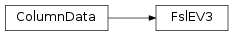# mvpa2.misc.fsl.base.FslEV3¶class `mvpa2.misc.fsl.base.``FslEV3`(source)

IO helper to read FSL’s EV3 files.

This is a three-column textfile format that is used to specify stimulation protocols for fMRI data analysis in FSL’s FEAT module.

Data is always read as `float`.

Attributes

 `durations` `intensities` `ncolumns` Returns the number of columns. `nevs` Returns the number of EVs in the file. `nrows` Returns the number of rows. `onsets`

Methods

 `clear`(() -> None.  Remove all items from D.) `copy`(() -> a shallow copy of D) `fromkeys`(...) v defaults to None. `get`((k[,d]) -> D[k] if k in D, ...) `get_ev`(evid) Returns a tuple of (onset time, simulus duration, intensity) for a certain EV. `has_key`((k) -> True if D has a key k, else False) `items`(() -> list of D’s (key, value) pairs, ...) `iteritems`(() -> an iterator over the (key, ...) `iterkeys`(() -> an iterator over the keys of D) `itervalues`(...) `keys`(() -> list of D’s keys) `pop`((k[,d]) -> v, ...) If key is not found, d is returned if given, otherwise KeyError is raised `popitem`(() -> (k, v), ...) 2-tuple; but raise KeyError if D is empty. `select_samples`(selection) Return new ColumnData with selected samples `setdefault`((k[,d]) -> D.get(k,d), ...) `to_events`(\*\*kwargs) Convert into a list of `Event` instances. `tofile`(filename) Write data to a FSL EV3 file. `update`(([E, ...) If E present and has a .keys() method, does: for k in E: D[k] = E[k] `values`(() -> list of D’s values) `viewitems`(...) `viewkeys`(...) `viewvalues`(...)

Read and write FSL EV3 files.

Parameters: source : str Filename of an EV3 file

Attributes

 `durations` `intensities` `ncolumns` Returns the number of columns. `nevs` Returns the number of EVs in the file. `nrows` Returns the number of rows. `onsets`

Methods

 `clear`(() -> None.  Remove all items from D.) `copy`(() -> a shallow copy of D) `fromkeys`(...) v defaults to None. `get`((k[,d]) -> D[k] if k in D, ...) `get_ev`(evid) Returns a tuple of (onset time, simulus duration, intensity) for a certain EV. `has_key`((k) -> True if D has a key k, else False) `items`(() -> list of D’s (key, value) pairs, ...) `iteritems`(() -> an iterator over the (key, ...) `iterkeys`(() -> an iterator over the keys of D) `itervalues`(...) `keys`(() -> list of D’s keys) `pop`((k[,d]) -> v, ...) If key is not found, d is returned if given, otherwise KeyError is raised `popitem`(() -> (k, v), ...) 2-tuple; but raise KeyError if D is empty. `select_samples`(selection) Return new ColumnData with selected samples `setdefault`((k[,d]) -> D.get(k,d), ...) `to_events`(\*\*kwargs) Convert into a list of `Event` instances. `tofile`(filename) Write data to a FSL EV3 file. `update`(([E, ...) If E present and has a .keys() method, does: for k in E: D[k] = E[k] `values`(() -> list of D’s values) `viewitems`(...) `viewkeys`(...) `viewvalues`(...)
`durations`
`get_ev`(evid)

Returns a tuple of (onset time, simulus duration, intensity) for a certain EV.

`intensities`
`nevs`

Returns the number of EVs in the file.

`onsets`
`to_events`(**kwargs)

Convert into a list of `Event` instances.

Parameters: kwargs Any keyword arugment provided would be replicated, through all the entries. Useful to specify label or even a chunk
`tofile`(filename)

Write data to a FSL EV3 file.